Courses

# Test: Electric Charges & Fields

## 20 Questions MCQ Test Physics Class 12 | Test: Electric Charges & Fields

Description
This mock test of Test: Electric Charges & Fields for JEE helps you for every JEE entrance exam. This contains 20 Multiple Choice Questions for JEE Test: Electric Charges & Fields (mcq) to study with solutions a complete question bank. The solved questions answers in this Test: Electric Charges & Fields quiz give you a good mix of easy questions and tough questions. JEE students definitely take this Test: Electric Charges & Fields exercise for a better result in the exam. You can find other Test: Electric Charges & Fields extra questions, long questions & short questions for JEE on EduRev as well by searching above.
QUESTION: 1

### Which of the following methods can be used to charge a metal sphere positively without touching it . Select the best

Solution:

This can be possible by induction, if a negative charge rod is placed near the sphere (at a distance not in touch) than it attracts positive charge inside the sphere towards its side and the negative charges collected on other side and when the surface of sphere connected to earth than the negative charge goes to earth and the sphere remain positively charged.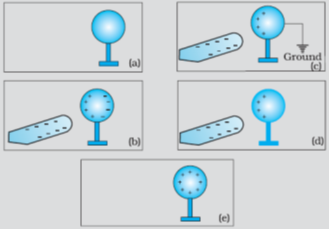QUESTION: 2

### When a negatively charged conductor is connected to earth,

Solution:

After earthing a positively charged conductor electrons flow from earth to conductor and if a negatively charged conductor is earthed then electrons flows from conductor to earth.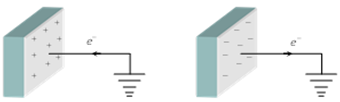QUESTION: 3

### A point charge of 2.0 μC is at the centre of a cubic Gaussian surface 9.0 cm on edge. What is the net electric flux through the surface?

Solution: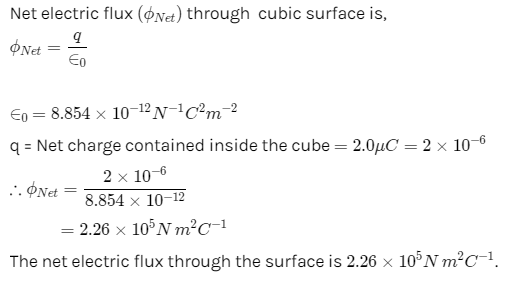QUESTION: 4

At any point on S on an electric field line:

Solution:

When a tangent is drawn at any point on field line then that tangent gives the direction of electric field at that point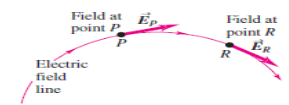QUESTION: 5

A point charge causes an electric flux of −1.0×103Nm2/C to pass through a spherical Gaussian surface of 10.0 cm radius centred on the charge.
(a) If the radius of the Gaussian surface were doubled, how much flux would pass through the surface?
(b) What is the value of the point charge?

Solution:

Electric flux is given by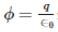since amount of charge not depends on size and shape so by making radius <  double the amount of charge remain same so electric flux remain same.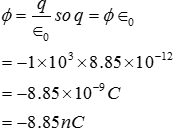QUESTION: 6

Electric charge:

Solution:

Electric charge, the basic property of matter carried by some elementary particles, such as atoms; ions; electrons; etc, that govern how the particles are affected by an electric or magnetic field.
Electric charge, which can be positive or negative, occurs in discrete natural units and is neither created nor destroyed.

QUESTION: 7

An electric dipole is

Solution:

An electric dipole is a separation of positive and negative charges. The simplest example of this is a pair of electric charges of equal magnitude but opposite sign, separated by some (usually small) distance. A permanent electric dipole is called an electret.

QUESTION: 8

A hollow spherical conductor of radius 2m carries a charge of 500 μ C. Then electric field strength at its surface is

Solution: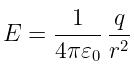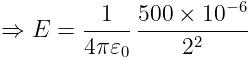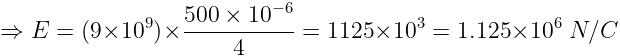QUESTION: 9

For forming an electric dipole between two point charges separated by a small distance, the two point charges are of

Solution:

An electric dipole is tagged as a pair of objects which possess equal & opposite charges, parted by a significantly small distance.

QUESTION: 10

The amount of work done in moving a charge from one point to another along an equipotential line or surface charge is

Solution:

Since Potential difference between two points in equipotential surfaces is zero , the work done between two points in equipotential surface is also zero .

QUESTION: 11

An electric field can deflect

Solution:

Only alpha rays are moving with small velocity and having charge so they will be affected by electric field.

QUESTION: 12

Under the influence of the coulomb field of charge +Q, a charge −q is moving around it in an elliptical orbit. Find out the correct statement(s).

Solution:

Since the charge –q is moving in elliptical orbit so to make its motion stable the total angular momentum of the charge is constant since it experience a centripetal force from the charge +Q so it follow the motion as the motion of earth around sun.

QUESTION: 13

Conservation of charges in tribo electric charging

Solution:

The triboelectric effect (also known as triboelectric charging) is a type of contact electrification in which certain materials become electrically charged after they come into frictional contact with a different material.
Example: Rubbing glass with fur, or a plastic comb through the hair.

When we charge something with static electricity, no electrons are made or destroyed. Electrons are just moved from one place to another. The net electric charge stays the same. This is called the principle of conservation of charge.
i.e. If a glass rod is rubbed with silk, some electrons moves from glass rod to silk no new charge is generated or destroyed.

QUESTION: 14

Consider a neutral conducting sphere. A positive point charge is placed outside the sphere. The net charge on the sphere is then,

Solution:

If a charge q is placed outside than the electric field lines incident on the conducting sphere , if some charge is developed due to thee lines than opposite surface becomes oppositely charge and the total charge becomes zero.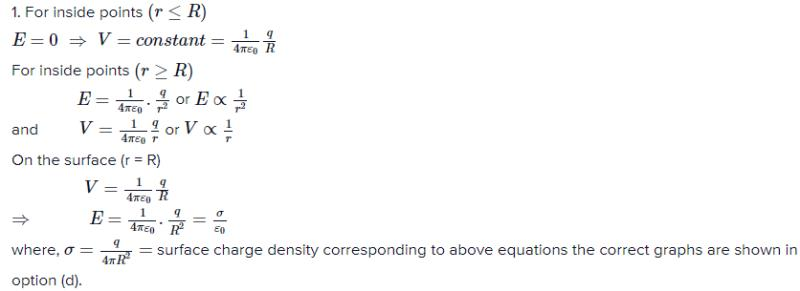QUESTION: 15

Two positive charges

Solution:

Acc to coulomb's law unlike charges always attract each other while like charges always repel each other.

QUESTION: 16

Ionization of a neutral atom is the

Solution:

It is not possible to remove or add protons/neutrons to an atom, but electrons can be added or removed by an atom easily.
By adding electrons it becomes negatively charged.
By removing electrons it becomes positively charged.

QUESTION: 17

A charge q is placed at the center of the line joining two equal charges Q. The system of the three charges will be in equilibrium if q is equal to

Solution: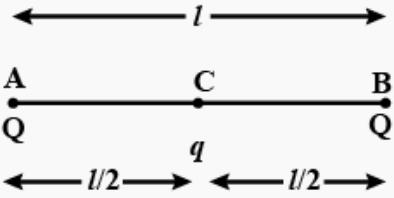Suppose in the following figure, equilibrium of charge B is considered. Hence for its equilibrium,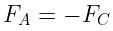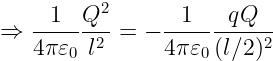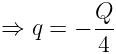QUESTION: 18

The electrostatic force is:

Solution:

The electrostatic force is an attractive and repulsive force between particles are caused due to their electric charges. The electric force between stationary charged bodies is conventionally known as the electrostatic force.

QUESTION: 19

Two charges A = -2.50 μC  and B = 6.0 μC are at a distance of 1 meter from each other. Distance from A at which the electric field is zero in meters is

Solution: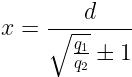where,
x = distance of the null point from the small charge
d = distance between two charges
q= bigger charge
q= small charge
+1 for like charges and -1 for unlike charges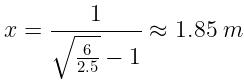QUESTION: 20

Conductors are materials

Solution:

Conductors are materials that permit electrons to flow freely from particle to particle. An object made of a conducting material will permit a charge to be transferred across the entire surface of the object.